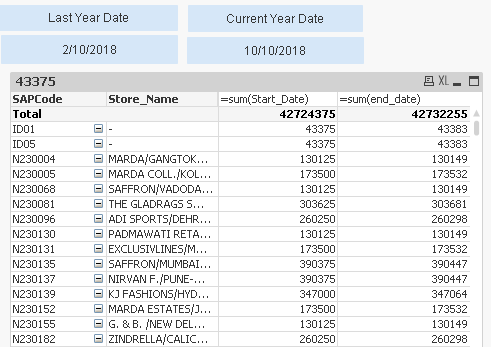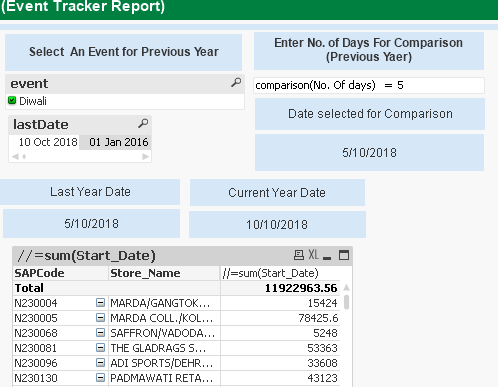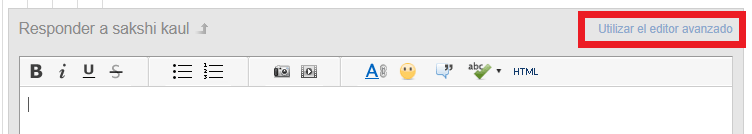New to QlikView

Discussion board where members can get started with QlikView.

Announcements
QlikWorld 2020: Join us May 11 - 14, 2020 in Phoenix, AZ. Register early and save \$400. Learn More
Contributor

how to write an expression for date range using variable

Hi,

I want to write an expression using variables start_date and end_date as shown in image

I want to show sales between the start_date and end_date. Both start_date and end_date are variables and these are text boxes

Now want to call both the variables in chart by writing expression using set analysis, Please help me in writing the same.14 RepliesMVP

Re: how to write an expression for date range using variable

Hi Sakshi, it can be something like:

Sum({<Start_Date={">=\$(vLastYearDate)<=\$(vCurrentYearDate)"}>} MetricField)

{<...>} -> Define set analysis

Start_Date= -> Field to filter

{"..."} -> Conditions to filter

\$() -> Gets the variable value

Contributor

Re: how to write an expression for date range using variable

Hi

This expression is working but in my report I have following requirement

I have an internal table maintained in the qlikview

InlinetableLastYear:

Date(Date#(InlineDate, 'DD/MM/YYYY'), 'D/MM/YYYY') as lastDate,

event

INLINE [

InlineDate,event

10/10/2018,Diwali

]
;

Now as shown in an image below , when the user will select comparison no. of days as 5

the date will be selected for which the user Is willing to see the sales

last_date=10 oct 2018 - comparison no of days =5

so final result is 5/10/2018

so here 5/10/2018 will become as start_date taken in variable in text box and end_date will be 10/10/2018so when I am changing the value of no of comparison days from 5 to 3 the date is changing in text box as seen in image but the result in chart is not being reflected so what changes are required in expression to achieve the same ?MVP

Re: how to write an expression for date range using variable

Following last example vCurrentYearDate can be "=lastDate"

And vLastYearDate can be "=Date('\$(vCurrentYearDate)'-\$(vComparisonDays)"

Sum({<Start_Date={">=\$(vLastYearDate)<=\$(vCurrentYearDate)"}>} MetricField)

Contributor

Re: how to write an expression for date range using variable

so you want me to do changes here ?

=Sum({<Sold_Date={">=\$("=Date('\$(vCurrentYearDate)'-\$(vComparisonDays)")<=\$("=lastDate)"}>} [inv_value])

so you want me to do the changes here?

please check the variable declaration list hereMVP

Re: how to write an expression for date range using variable

can you upload a sample copy of the document?

In file -> Reduce data you can make a smaller document.

In Document properties -> Cipher you can scramble field values

Contributor

Re: how to write an expression for date range using variable

now from where I can get attach option to attach a doucument?MVP

Re: how to write an expression for date range using variableBelow the body you have the option to 'attach'

Contributor

Re: how to write an expression for date range using variable

can you please send me the link from where I will get this advanced editor page ?

Contributor

Re: how to write an expression for date range using variableWhy I am not able to attach this document ?